# Mass, atomic and empirical formulasPage 1

#### WATCH ALL SLIDES

Slide 1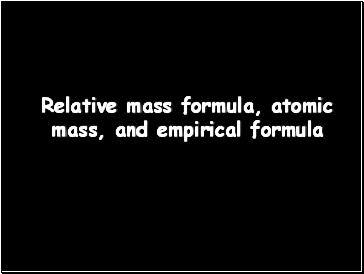Relative mass formula, atomic mass, and empirical formula

Slide 2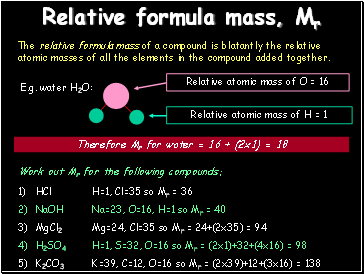## Relative formula mass, Mr

The relative formula mass of a compound is blatantly the relative atomic masses of all the elements in the compound added together.

E.g. water H2O:

Therefore Mr for water = 16 + (2x1) = 18

Work out Mr for the following compounds:

HCl

NaOH

MgCl2

H2SO4

K2CO3

H=1, Cl=35 so Mr = 36

Na=23, O=16, H=1 so Mr = 40

Mg=24, Cl=35 so Mr = 24+(2x35) = 94

H=1, S=32, O=16 so Mr = (2x1)+32+(4x16) = 98

K=39, C=12, O=16 so Mr = (2x39)+12+(3x16) = 138

Slide 3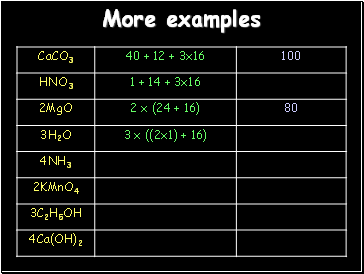## More examples

Slide 4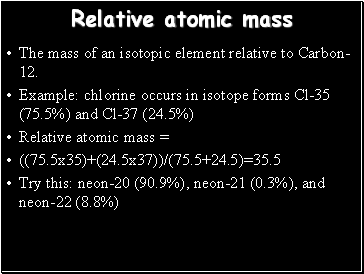## Relative atomic mass

The mass of an isotopic element relative to Carbon-12.

Example: chlorine occurs in isotope forms Cl-35 (75.5%) and Cl-37 (24.5%)

Relative atomic mass =

((75.5x35)+(24.5x37))/(75.5+24.5)=35.5

Try this: neon-20 (90.9%), neon-21 (0.3%), and neon-22 (8.8%)

Slide 5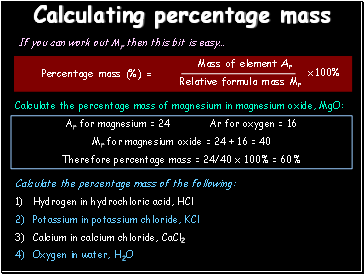## Calculating percentage mass

If you can work out Mr then this bit is easy…

Calculate the percentage mass of magnesium in magnesium oxide, MgO:

Ar for magnesium = 24 Ar for oxygen = 16

Mr for magnesium oxide = 24 + 16 = 40

Therefore percentage mass = 24/40 x 100% = 60%

Calculate the percentage mass of the following:

Hydrogen in hydrochloric acid, HCl

Potassium in potassium chloride, KCl

Calcium in calcium chloride, CaCl2

Oxygen in water, H2O

Slide 6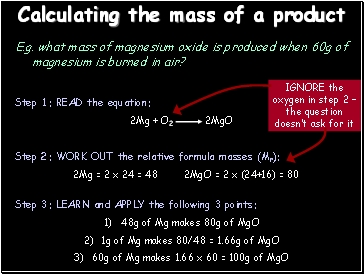## Calculating the mass of a product

E.g. what mass of magnesium oxide is produced when 60g of magnesium is burned in air?

Step 3: LEARN and APPLY the following 3 points:

48g of Mg makes 80g of MgO

1g of Mg makes 80/48 = 1.66g of MgO

60g of Mg makes 1.66 x 60 = 100g of MgO

Step 2: WORK OUT the relative formula masses (Mr):

2Mg = 2 x 24 = 48 2MgO = 2 x (24+16) = 80

Slide 7Work out Mr: 2H2O = 2 x ((2x1)+16) = 36 2H2 = 2x2 = 4

36g of water produces 4g of hydrogen

So 1g of water produces 4/36 = 0.11g of hydrogen

6g of water will produce (4/36) x 6 = 0.66g of hydrogen

Mr: 2Ca = 2x40 = 80 2CaO = 2 x (40+16) = 112

80g produces 112g so 10g produces (112/80) x 10 = 14g of CaO

Mr: 2Al2O3 = 2x((2x27)+(3x16)) = 204 4Al = 4x27 = 108

204g produces 108g so 100g produces (108/204) x 100 = 52.9g of Al2O3

Slide 8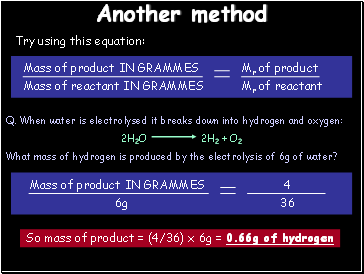Go to page:
1  2